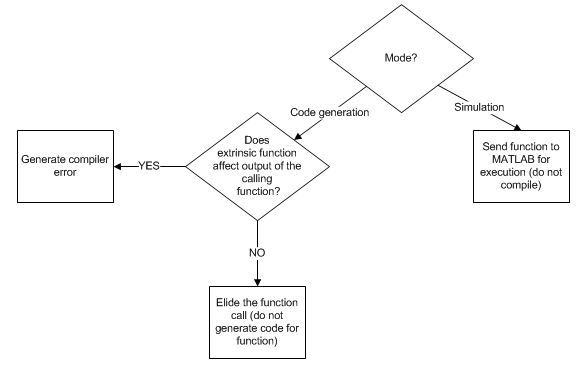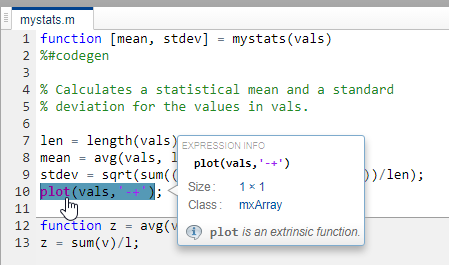## Use MATLAB Engine to Execute a Function Call in Generated Code

When processing a call to a function `foo` in your MATLAB® code, the code generator finds the definition of `foo` and generates code for its body. In some cases, you might want to bypass code generation and instead use the MATLAB engine to execute the call. Use `coder.extrinsic('foo')` to declare that calls to `foo` do not generate code and instead use the MATLAB engine for execution. In this context, `foo` is referred to as an extrinsic function. This functionality is available only when the MATLAB engine is available during execution. Examples of such situations include execution of MEX functions, Simulink® simulations, or function calls at the time of code generation (also known as compile time).

If you generate standalone code for a function that calls `foo` and includes `coder.extrinsic('foo')`, the code generator attempts to determine whether `foo` affects the output. If `foo` does not affect the output, the code generator proceeds with code generation, but excludes `foo` from the generated code. Otherwise, the code generator produces a compilation error.

Including the `coder.extrinsic('foo')` directive inside a certain MATLAB function declares all calls to `foo` inside that MATLAB function as extrinsic. Alternatively, you might want to narrow the scope of extrinsic declaration to just one call to `foo`. See Call MATLAB Functions Using feval (MATLAB Coder).### When To Declare a Function as Extrinsic

These are some common situations in which you might consider declaring a MATLAB function as extrinsic:

• The function performs display or logging actions. Such functions are useful primarily during simulation and are not used in embedded systems.

• In your MEX execution or Simulink simulation, you want to use a MATLAB function that is not supported for code generation. This workflow does not apply to non-simulation targets.

• You instruct the code generator to constant fold a function call by using `coder.const`. In such situations, the function is called only during code generation when the MATLAB engine is available for executing the call.

### Use the `coder.extrinsic` Construct

To declare a function `foo` as extrinsic, include this statement in your MATLAB code.

`coder.extrinsic('foo')`

When declaring functions as extrinsic for code generation, adhere to these rules:

• Declare the function as extrinsic before you call it.

• Do not use the extrinsic declaration in conditional statements.

• Assign the return value of an extrinsic function to a known type. See Working with mxArrays (MATLAB Coder).

For additional information and examples, see `coder.extrinsic`.

The code generator automatically treats many common MATLAB visualization functions, such as `plot`, `disp`, and `figure`, as extrinsic. You do not have to explicitly declare them as extrinsic functions by using `coder.extrinsic`. For example, you might want to call `plot` to visualize your results in the MATLAB environment. If you generate a MEX function from a function that calls `plot`, and then run the generated MEX function, the code generator dispatches calls to the `plot` function to the MATLAB engine. If you generate a library or executable, the generated code does not contain calls to the `plot` function.

If you generate MEX or standalone C/C++ code by using MATLAB Coder™, the code generation report highlights calls from your MATLAB code to extrinsic functions. By inspecting the report, you can determine which functions are supported only in the MATLAB environment.#### Scope of Extrinsic Function Declarations

The `coder.extrinsic` construct has function scope. For example, consider the following code:

```function y = foo %#codegen coder.extrinsic('rat','min'); [N D] = rat(pi); y = 0; y = min(N, D); ```

In this example, `rat` and `min` as treated as extrinsic every time they are called in the main function `foo`. There are two ways to narrow the scope of an extrinsic declaration inside the main function:

• Declare the MATLAB function extrinsic in a local function, as in this example:

```function y = foo %#codegen coder.extrinsic('rat'); [N D] = rat(pi); y = 0; y = mymin(N, D); function y = mymin(a,b) coder.extrinsic('min'); y = min(a,b); ```

Here, the function `rat` is extrinsic every time it is called inside the main function `foo`, but the function `min` is extrinsic only when called inside the local function `mymin`.

• Instead of using the `coder.extrinsic` construct, call the MATLAB function using `feval`. This approach is described in the next section.

#### Extrinsic Declaration for Nonstatic Methods

Suppose that you define a class `myClass` that has a nonstatic method `foo`, and then create an instance `obj` of this class. If you want to declare the method `obj.foo` as extrinsic in your MATLAB code that you intend for code generation, follow these rules:

• Write the call to `foo` as a function call. Do not write the call by using the dot notation.

• Declare `foo` to be extrinsic by using the syntax `coder.extrinsic('foo')`.

For example, define `myClass` as:

```classdef myClass properties prop = 1 end methods function y = foo(obj,x) y = obj.prop + x; end end end ```

Here is an example MATLAB function that declares `foo` as extrinsic.

```function y = myFunction(x) %#codegen coder.extrinsic('foo'); obj = myClass; y = foo(obj,x); end ```

Nonstatic methods are also known as ordinary methods. See Method Syntax.

Use the `coder.extrinsic` construct to:

• Call MATLAB functions that do not produce output during simulation without generating unnecessary code.

• Make your code self-documenting and easier to debug. You can scan the source code for `coder.extrinsic` statements to isolate calls to MATLAB functions, which can potentially create and propagate `mxArrays`. See Working with mxArrays (MATLAB Coder).

### Call MATLAB Functions Using `feval`

To narrow the scope of extrinsic declaration to just one function call, use the function `feval`. `feval` is automatically interpreted as an extrinsic function during code generation. So, you can use `feval` to call functions that you want to execute in the MATLAB environment, rather than compile to generated code.

Consider this example:

```function y = foo coder.extrinsic('rat'); [N D] = rat(pi); y = 0; y = feval('min',N,D); ```

Because `feval` is extrinsic, the statement `feval('min',N,D)` is evaluated by MATLAB, not compiled, which has the same result as declaring the function `min` extrinsic for just this one call. By contrast, the function `rat` is extrinsic throughout the function `foo`.

The code generator does not support the use of `feval` to call local functions or functions that are located in a private folder.

### Working with mxArrays

The run-time output of an extrinsic function is an `mxArray`, also known as a MATLAB array. The only valid operations for `mxArrays` are:

• Storing an `mxArray` in a variable.

• Passing an `mxArray` to an extrinsic function.

• Returning an `mxArray` from a function back to MATLAB.

• Converting an `mxArray` to a known type at run time. Assign the `mxArray` to a variable whose type is already defined by a prior assignment. See the following example.

To use an `mxArray` returned by an extrinsic function in other operations (for example, returning it from a MATLAB Function block to Simulink execution), you must first convert it to a known type.

If the input arguments of a function are `mxArrays`, the code generator automatically treats the function as extrinsic.

#### Convert mxArrays to Known Types

To convert an `mxArray` to a known type, assign the `mxArray` to a variable whose type is defined. At run time, the `mxArray` is converted to the type of the variable that it is assigned to. If the data in the `mxArray` is not consistent with the type of the variable, you get a run-time error.

For example, consider this code:

```function y = foo %#codegen coder.extrinsic('rat'); [N D] = rat(pi); y = min(N,D); ```

Here, the top-level function `foo` calls the extrinsic MATLAB function `rat`, which returns two `mxArrays` representing the numerator `N` and denominator `D` of the rational fraction approximation of `pi`. You can pass these `mxArrays` to another MATLAB function, in this case, `min`. Because the inputs passed to `min` are `mxArrays`, the code generator automatically treats `min` as an extrinsic function. As a result, `min` returns an `mxArray`.

While generating a MEX function by using MATLAB Coder, you can directly assign this `mxArray` returned by `min` to the output `y` because the MEX function returns its output to MATLAB.

`codegen foo`
`Code generation successful.`

But if you put `foo` in a MATLAB Function block in a Simulink model and then update or run the model, you get this error:

```Function output 'y' cannot be an mxArray in this context. Consider preinitializing the output variable with a known type. ```

This error occurs because returning an `mxArray` back to Simulink is not supported. To fix this issue, define `y` to be the type and size of the value that you expect `min` to return, in this case, a scalar double:

```function y = foo %#codegen coder.extrinsic('rat'); [N D] = rat(pi); y = 0; % Define y as a scalar of type double y = min(N,D);```

In this example, the output of the extrinsic function `min` affects the output `y` of the entry-point function `foo` for which you are generating code. If you attempt to generate standalone code (for example, a static library) for `foo`, the code generator is unable to ignore the extrinsic function call and produces a code generation error.

`codegen -config:lib foo`
```??? The extrinsic function 'min' is not available for standalone code generation. It must be eliminated for stand-alone code to be generated. It could not be eliminated because its outputs appear to influence the calling function. Fix this error by not using 'min' or by ensuring that its outputs are unused. Error in ==> foo Line: 4 Column: 5 Code generation failed: View Error Report Error using codegen```

### Restrictions on Using Extrinsic Functions

The full MATLAB run-time environment is not supported during code generation. Therefore, the following restrictions apply when calling MATLAB functions extrinsically:

• Some MATLAB functions that inspect the caller, or that read or write to the caller workspace, are not supported for code generation. Such functions include:

• Functions in generated code can produce unpredictable results if your extrinsic function performs these actions at run time:

• Changes folders

• Changes the MATLAB path

• Deletes or adds MATLAB files

• Changes warning states

• Changes MATLAB preferences

• The code generator does not support the use of `coder.extrinsic` to call functions that are located in a private folder.
• The code generator does not support the use of `coder.extrinsic` to call local functions.